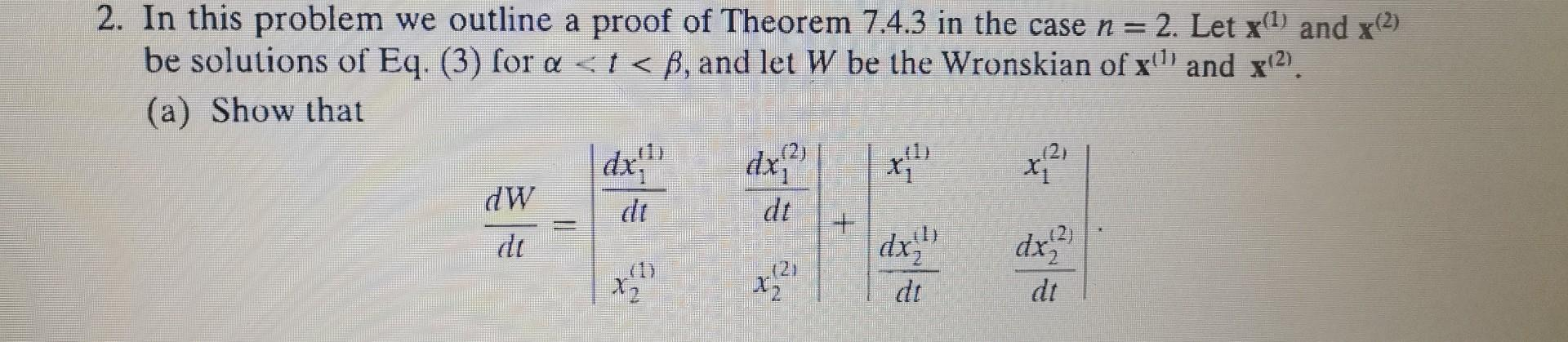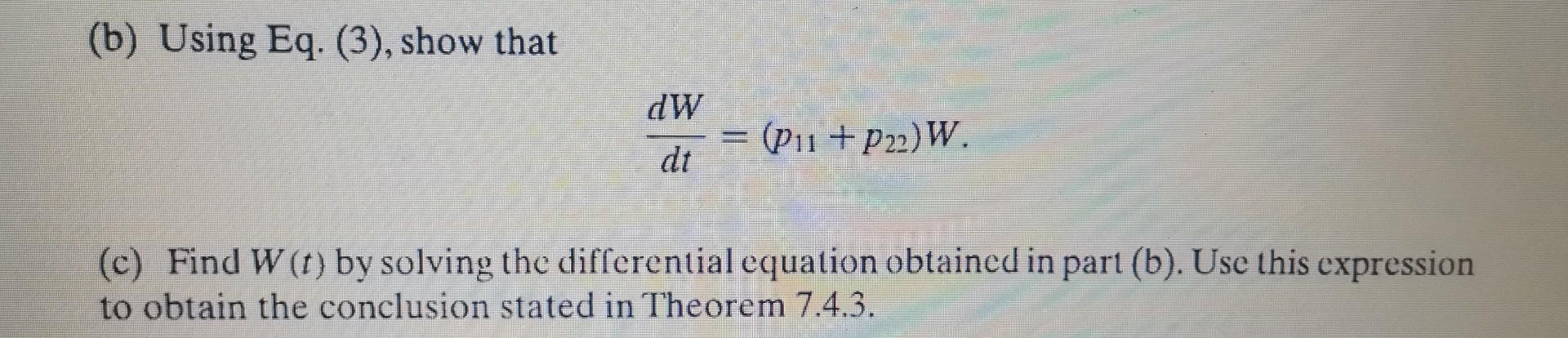# (Solved): 2. In this problem we outline a proof of Theorem 7.4.3 in the case n=2. Let x(1) and x(2) b ...2. In this problem we outline a proof of Theorem 7.4.3 in the case . Let and be solutions of Eq. (3) for , and let be the Wronskian of and . (a) Show that (b) Using Eq. (3), show that (c) Find by solving the differential equation obtained in part (b). Use this expression to obtain the conclusion stated in Theorem 7.4.3.

We have an Answer from Expert